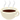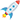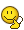# Speed of Light: Spaceship & Gravity Law Impact

• I
As I said in the last paragraph of the post you quoted, relativistic mass is not a source of gravity. It is the stress-energy tensor that is the source of gravity, so relativistic mass is not relevant to your scenario.f

A ship that moves at a speed very close to light, its relative mass increases and may be comparable to the mass of the planets or the sun, in this case it can change the orbit of the planets and the sun (according to the law of gravity of two bodies)
Is this analysis correct?

No. Consider the view from the spaceship - it is at rest and the solar system is moving fast. From this perspective there is no reason for the orbits to change.

More generally, "relativistic mass" is a deprecated concept precisely because of this kind of confusion. The source of gravity in relativity is the stress-energy tensor, not the relativistic mass.

•vanhees71, Dale, Orodruin and 1 other person
More generally, "relativistic mass" is a deprecated concept precisely because of this kind of confusion.

Also note that Newtonian gravity with instant action at a distance is fundamentally incompatible with special relativity. It should therefore cone as no surprise that it needs modification to include relativistic effects.

Last edited:
•vanhees71 and Ibix
No. Consider the view from the spaceship - it is at rest and the solar system is moving fast. From this perspective there is no reason for the orbits to change.

More generally, "relativistic mass" is a deprecated concept precisely because of this kind of confusion. The source of gravity in relativity is the stress-energy tensor, not the relativistic mass.
From the reference frame of the star, the spacecraft has a lot of mass, so it must interact gravitationally.

•PeroK and weirdoguy
From the reference frame of the star, the spacecraft has a lot of mass, so it must interact gravitationally.
No. The aim of the first paragraph in the post you quoted was to point out a contradiction in your thinking. Stated plainly, relativistic mass cannot be a source of gravity because there are some frames where the relativistic mass is low and some where it is high. If it were a source of gravity then this would lead to different frames having inconsistent predictions of gravitational effects.

As I said in the last paragraph of the post you quoted, relativistic mass is not a source of gravity. It is the stress-energy tensor that is the source of gravity, so relativistic mass is not relevant to your scenario.

Last edited:
•malawi_glenn
From the reference frame of the star, the spacecraft has a lot of mass, so it must interact gravitationally.
No, you are simply wrong in trying to apply Newtonian gravity in a relativistic setting. Please read the resources pointed out to you.

•From the reference frame of the star, the spacecraft has a lot of mass, so it must interact gravitationally.
And along the same line of thought, from the reference frame of the ship, the star will have gained mass, so the ship is attracted to it more. Depending on who's looking, either the ship would start orbiting the star or the star would orbit the ship. Both can't be true at the same time.

In relativity, there are more components to the source of gravity (the aforementioned stress-energy tensor) than just the energy density (=relativistic mass). While the energy component increases effective gravity, others can decrease it. Together they 'conspire' to cancel out so that the dynamics of a system doesn't change just because you change the reference frame.
What you are doing in your thought process here, is take one component of the relativistic source of gravity, and treat it as if it were a complete replacement for the source of gravity in Newtonian mechanics.
When relative velocities are low, this a sensible approximation; then the gamma factor is negligible and you have just your regular Newtonian gravity with rest mass dominating all other effects.
But when relative velocities are high enough to start worrying about relativistic increase in energy density, you can no longer ignore the remaining components and expect an answer that will not produce paradoxes.

•malawi_glenn
In SR, there is a simple, frame independent way to look at this. In SR, there is the notion of invariant mass. The invariant mass of the rocket never changes, no matter what its velocity relative to the solar system is. Similarly, the invariant mass of the solar system as a whole never changes, no matter what the relative velocity of rocket and sun are.

However, there is a nuance here. The invariant mass of the system of solar system plus rocket can be made arbitrarily large as their relative velocity increases arbitrarily close to c (speaking of SR only). Note that this is a 'mass' of the system as a whole, not attributable to the rocket or the solar system. Note also, that this 'system invariant mass' is unchanged (in SR) whether the rocket is far away from the solar system or inside it. But the 'invariant energy density' peaks when the rocket is inside the solar system. But ...

to discuss gravity, we need GR rather than SR. There is no simple analog of invariant mass in GR. However, if one assumes an empty universe with no dark energy, just the solar system and rocket, there are GR arguments that suggest as the rocket and solar system approach each other, they can collapse into a BH. This would be a collective interaction, not attributable to any component, and it would involve the whole system - there would be one large BH formed. The relevant notion in GR is the 'hoop conjecture'.

Last edited:
The invariant mass of the system of solar system plus rocket can be made arbitrarily large as their relative velocity increases arbitrarily close to c (speaking of SR only). Note that this is a 'mass' of the system as a whole, not attributable to the rocket or the solar system.
In SR context, why is it the case ? Thanks.

In SR context, why is it the case

Just compute ## \sqrt{ (E_\text{sun}+ E_\text{rocket})^2 - |\vec p_\text{sun} + \vec p_\text{rocket}|^2 }## You can make ##\vec p _\text{rocket}## arbitrarily large since ##\vec p _\text{rocket} = \gamma (v_\text{rocket})m_\text{rocket} \vec v_\text{rocket} ##

Last edited by a moderator:
Just compute ## \sqrt{ M_\text{sun}+ m_\text{sun} - |\vec p_\text{sun} + \vec p_\text{rocket}| }##
##m_\text{sun}## should read the rest mass of the rocket, right ?

•malawi_glenn
##m_\text{sun}## should read the rest mass of the rocket, right ?
Yup, I fixed that typo. Need more coffeeI found another error... the M and m should be energies...This is better actually (invariant mass squared)
##(m_\text{sun})^2 + (m_\text{rocket})^2 + 2\left( E_\text{sun}E_\text{rocket} - \vec{p}_\text{sun} \cdot \vec{p}_\text{rocket} \right)##

Now, if sun is basically at rest, and Erocket >> msun >> mrocket this becomes
## \sim 2 m_\text{sun}E_\text{rocket} ##

Just compute ## \sqrt{ E_\text{sun}+ E_\text{rocket} - |\vec p_\text{sun} + \vec p_\text{rocket}| }## You can make ##\vec p _\text{rocket}## arbitrarily large since ##\vec p _\text{rocket} = \gamma (v_\text{rocket})m_\text{rocket} \vec v_\text{rocket} ##
## \sqrt{ (E_\text{sun}+ E_\text{rocket})^2 - (\vec p_\text{sun} + \vec p_\text{rocket})^2 }##

•malawi_glenn
## \sqrt{ (E_\text{sun}+ E_\text{rocket})^2 - (\vec p_\text{sun} + \vec p_\text{rocket})^2 }##
I needed two cups of coffee this morning=## \sqrt{ (E_\text{sun}+ E_\text{rocket})^2 - (\vec p_\text{sun} + \vec p_\text{rocket})^2 }##
This is the value of 'system rest/invariant mass' that whatever inertial frame assigns to the system considered, right ?

•malawi_glenn
This is the value of 'system rest/invariant mass' that whatever inertial frame assigns to the system considered, right ?
Yes, the value of this is independent of which Lorentz-frame you calculate it in.

•cianfa72